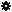﻿ Object Model: Edge.NeighborEdges

Edge.NeighborEdges

## Edge.NeighborEdgesv3.0

### Description

Returns a collection of Edge objects for all edge neighbors within a given distance. If the distance is 1 the Edge objects are returned in counter-clockwise order.

### C# Syntax

 ```EdgeCollection Edge.NeighborEdges( Int32 in_lDistance ); ```

### Scripting Syntax

 ```oReturn = Edge.NeighborEdges( [Distance] ); ```

### Parameters

Parameter Type Description
Distance Integer Distance is an integer value representing the degree of neighborhood (for example, degree=2 for an edge means its adjacent edges plus the adjacent edges of the adjacent edges).

Default Value: 1

### Examples

#### VBScript Example

 ```set oCube = ActiveSceneRoot.AddGeometry( "Cube", "MeshSurface" ) set oGeometry = oCube.ActivePrimitive.Geometry for each Edge in oGeometry.Edges set oNeighbors = Edge.NeighborEdges str = "Edges neighbors of edge( " & Edge.index & ") are :" for each n in oNeighbors str = str & " " & n.index next logmessage str next ```